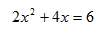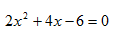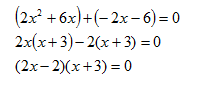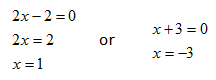Solving

When solving polynomials, it is helpful to turn the polynomial into its linear factors. This can be accomplished by dividing, as shown in the last lesson, or by factoring. If the polynomial cannot be solved using either of these ways, it is sometimes helpful to use the quadratic formula.

Example 1 Solve the following polynomial:.

Step 1. Set the equation equal to zero.Step 2. Factor, if possible.Step 3. Use the Zero Product Property to set each expression equal to zero and solve.*Note: This problem could have been solved by first factoring out the GCF.